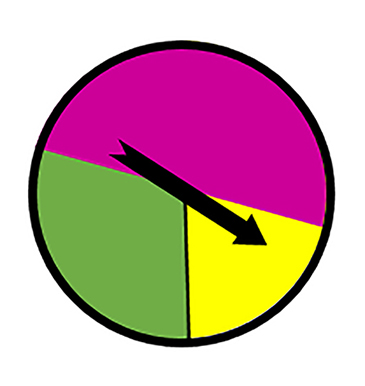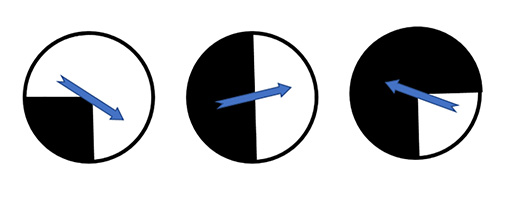Education & Development

### Become an OU studentTeaching mathematics

Start this free course now. Just create an account and sign in. Enrol and complete the course for a free statement of participation or digital badge if available.

# 4.4 Spinners

The probability of landing on a particular colour on a spinner is determined by the number of sections rather than the size of the angles.Figure 32 A spinner

When looking at spinners, it is important to look at the size of the sectors. In Figure 32 there are 3 possible outcomes but the angle for the green section is much larger than for the yellow section, so the probability of landing on green is much higher.

## Activity 16 True or false?

Timing: Allow 5 minutes

Read each of the statements below and decide whether they are true or false. Give reasons for your decision and try to explain the misconception you think each learner has formed.

(a) I flipped a coin 3 times and got 3 heads. It is more likely to be tails than heads if I spin it again.

(b) I tossed a coin 8 times and got tails every time. That proves that this coin must be biased as half of the time I should have got heads.

(c) I roll two dice and add the results. The probability of getting a total of 6 is because there are 12 different possibilities and 6 is one of them.

(d) If six dice are thrown at the same time, I am less likely to get 1, 1, 1, 1, 1, 1 than 1, 2, 3, 4, 5, 6.

(e) I spin two coins. The probability of getting heads and tails is because I can get heads and heads, head and tails, or tails and tails.

(f) Each spinner has two sections – one black and one white. The probability of getting black is for each spinner.Figure 33 Three spinners
To use this interactive functionality a free OU account is required. Sign in or register.
Interactive feature not available in single page view (see it in standard view).

### Discussion

(a) False.

The probability remains .

(b) False.

It could be a biased coin but the point is, this is not enough evidence to prove that it is. If it were rolled 100 times with only tails appearing, that would give a fair indication that the coin is biased. However, it is possible to get tails 8 times in a row, so it is not enough in itself to prove that the coin is biased.

(c) False.

First, the sample space is the totals which can be made from two dice. Totals can be made from 2 to 12 (1 is not possible with two dice). Second, the probability of each score is not equal. For instance, 7 can be made in 6 different ways: 1 + 6, 2 + 5, 3 + 4, 4 + 5, 5 + 2, 6 + 2, whereas 2 can only be made in 1 way: 1 + 1.

The probability of getting a 6 is .

(d) False.

Both outcomes are equally likely. The sample space would be all of the possible combinations. So 1, 1, 1, 1, 1, 1 is no less likely than 1, 2, 3, 4, 5, 6.

Of course, if you are not worried about the order (i.e. you can have the numbers 1 to 6 in any order) then that outcome is more likely than 1, 1, 1, 1, 1, 1 as there is only one arrangement of this but the numbers 1 to 6 can be ordered in 6 × 5 × 4 × 3 × 2 × 1 ways.

(e) False.

We discussed this example earlier. Yes, there are three outcomes if you don’t consider the order, but there are two ways to get heads, tails – HT and TH – so the probability of getting heads, tails is .

(f) False.

In the first spinner in Figure 33 the probability of landing on black is as half of the spinner is shaded black.

In the second spinner, the probability of landing on black is only .

In the third spinner the probability of landing on black is .

It is important to take the proportion into consideration, not just how many different outcomes there are.